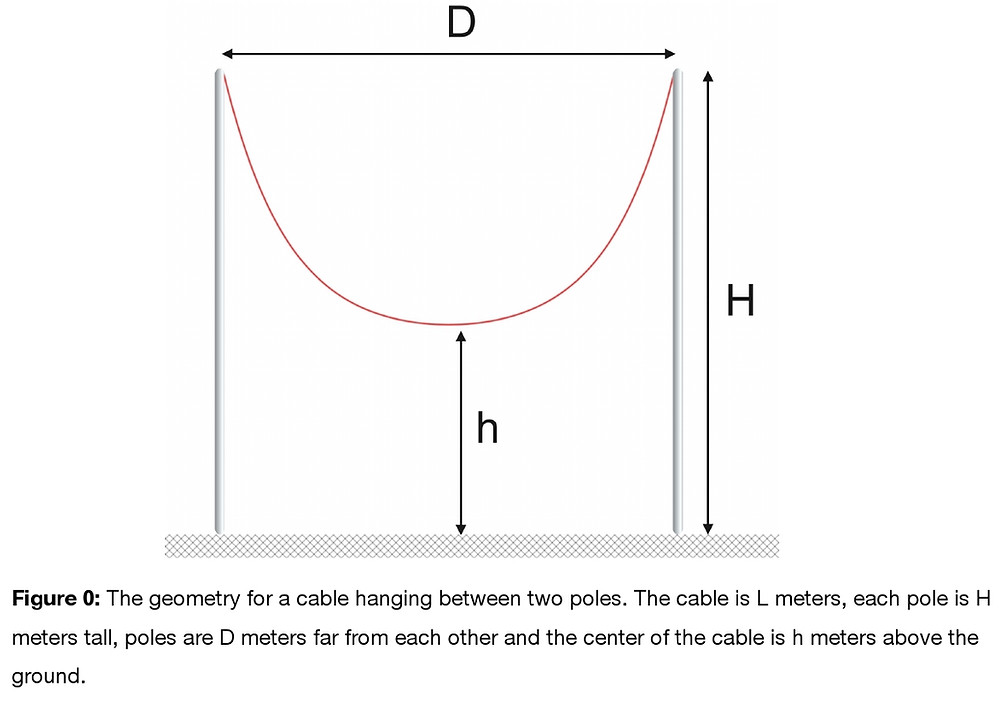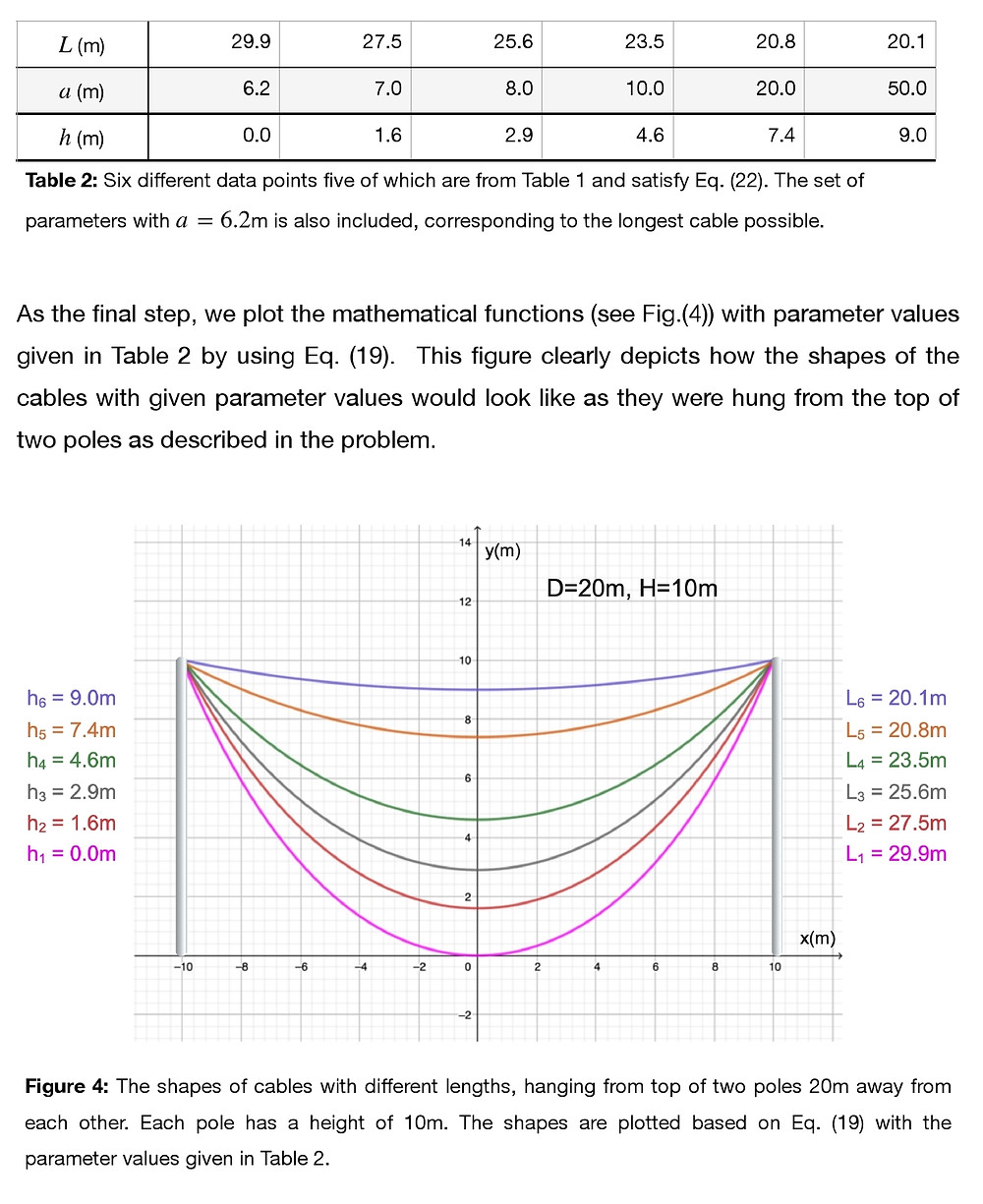Search

# Mathematical Shape of a Hanging Cable from Two Poles

Updated: Mar 17

A cable with a length of L meters and a mass of M kilograms is hanging from the top of two poles that are both H meters tall. The poles are D meters far from each other. If the center of the cable is h meters above the ground and the cable has uniform mass density throughout its length (see the below picture),

a) What will be the mathematical function which describes the shape of the cable?

b) Find an expression for the tension at the mid-point (center) of the cable in terms of the parameters given in the problem.

c) Suppose two poles each with a height of 10m are 20m apart from each other; i.e. D=20m and H=10m. Determine the value of h for cables (with different lengths) hanging from the top of these two poles and plot the mathematical functions which represent the shapes for the cables chosen. For any cable, no more than one point (which is the mid-point of the cable) can touch the ground.

d) For a given D and H values, the longest cable can determined as the one whose lowest point (i.e. the mid-point) touches the ground (h=0). In order to maintain the shape given by the mathematical function found in Part c, we require that no other point touch the ground other than the mid-point. By varying D and H values, show how the length of the longest cable changes.## SOLUTION:## Recent Posts

See All

#### PARTICLE TRAJECTORY IN E&M FIELDS